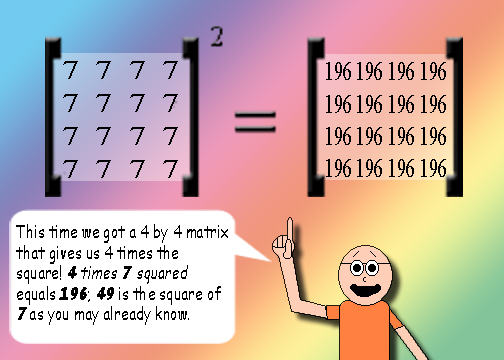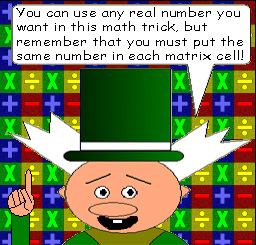# Squaring Square Matrices

## Example:## Here's what happens with 3 by 3 matrices:## Here's a 4 by 4 matrix:I bet you can guess what will happen with a 5 by 5 matrix!

## There's a function for this math trick. The function is:

y = zx2

x = the number in each matrix cell

y = the number of rows & columns multiplied by the square of the number in each matrix cell

z = the number of rows & columns

## Y is the number you'll get in each cell of the equation matrix!